# Examples of equations for 5th grade (10y)

#### Number of problems found: 104

• Equation with one variableSolve the following equation with one unknown: 5(7s + 5) =130
• If-then equationIf 5x - 17 = -x + 7, then x =
• Eq1Solve equation: 4(a-3)=3(2a-5)
• Equation algebraogram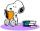Solve the equation: oco + ivo = cita How much has the task of solutions?
• Unknown number xyzFind the number that its triple is 24. Solve by equation.
• Star equationWrite digits instead of stars so that the sum of the written digits is odd and is true equality: 42 · ∗8 = 2 ∗∗∗
• Six te 2If 3t-7=5t, then 6t=
• Cakes1/3 poppy cake, 1/3 apple, 15 pieces of cheese. How many are totally cakes?
• Brother and sisterWhen I was 8, my sister was 4, and now I am 18. How old is my sister?
• Expression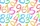If A=2 B=3 evaluate expression A(B+A) and multiply it by A
• I thinkI think the number. If I add the number 983 to it and divide it by 6, I get the number 1428. What number do I think?
• NumberDetermine unknown number if you know that difference between five times and triple of number is 42.
• I thinkI think a number. When I multiply it by five, and after that I subtract 477, I get the same number as if I multiplied it twice. What number do I think?
• FamilyThe twins Nina and Ema have five years younger brother Michal. Together have 43 years. How old is Michal?
• Identity123456789 = 100 Use only three plus or minus characters to correct previous identity/equation.
• Father and sons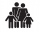Father is 27 and his sons 2 and one year. In how many years will his sons sum up to half his age?
• Seven timesWhich number seven times is just as higher as 27, how much is smaller than 29?
• Unknown numberI think the number: Its half is 16 bigger than his quarter. I think the number N . ..
• Jam cakes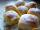Mom baked a third of plum jam cakes, one third cheesecakes and 18 poppy. How many cakes she had bake?
• Ring The Bell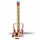Lucy and David challenged each other at the “Ring The Bell” game. David scored 120 points, although Lucy and David scored 235 points. How many points did Lucy score? Draw a diagram and equation to represent this situation. Use ‘L’ as the missing var.

Do you have an interesting mathematical word problem that you can't solve it? Submit a math problem, and we can try to solve it.

We will send a solution to your e-mail address. Solved examples are also published here. Please enter the e-mail correctly and check whether you don't have a full mailbox.

Please do not submit problems from current active competitions such as Mathematical Olympiad, correspondence seminars etc...

Do you have a linear equation or system of equations and looking for its solution? Or do you have a quadratic equation? Equations Math problems. Examples for 5th grade (the fifth graders).